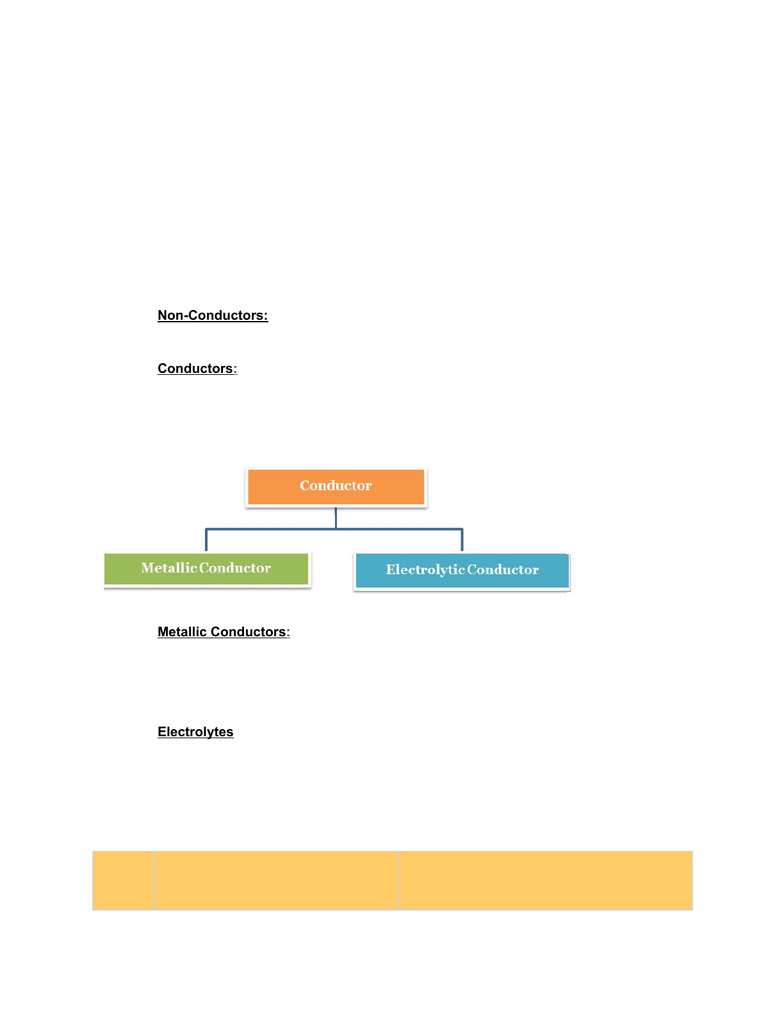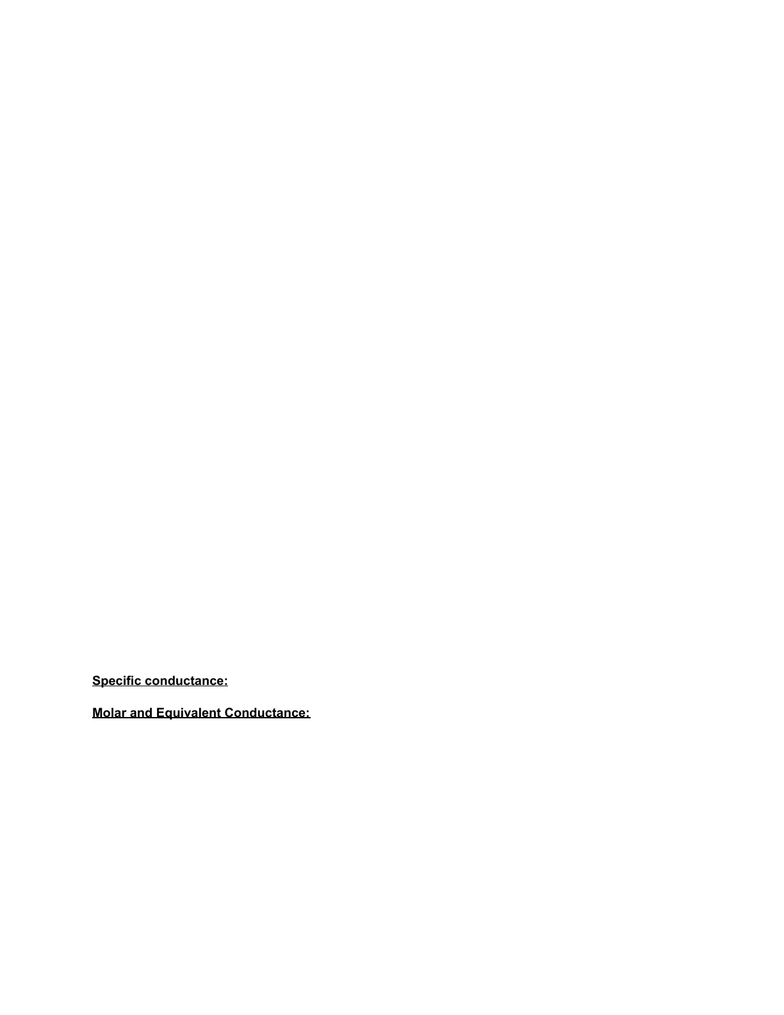# CHE 101 Chapter Notes - Chapter 12: Redox, Electrochemistry, Electric Current

0 views13 pages
School
Department
Course
ProfessorRevision Notes on Redox Reactions and Electrochemistry:
Electrochemistry is the branch of chemistry which deals with the chemical changes caused in the
matter by passage of electric current and conversion of chemical energy into electrical energy and
vice versa.
Conductors and Non Conductors
Substances around us can be divided into two classes based on their ability of conduct electricity:
Non-Conductors: Those substances which do not allow electric current to pass through
them are called non-conductors or insulators. Example: - wood, plastic glass, rubber etc.
Conductors: Those substances which allow electric current to flow through tem are
called conductors. Examples: Copper, Iron, Gold, Silver, Graphite, salt solution etc.
Conductors can further be divided into two groups
Metallic Conductors: These conductors conduct electricity or electric current by
movement of electrons without undergoing any chemical change during the process.
These conduct electricity in both solid as well as molten state. Example: All the metals
and Graphite
Electrolytes: Those substances which conduct electricity only when they are present in
aqueous solution and not in solid form are called electrolytes. These conduct electricity by
movement of ions in solutions.
Comparison of Electrolytic and Metallic Conduction
S.No
Metallic Conduction
Electrolytic Conduction
Unlock document

This preview shows pages 1-3 of the document.
Unlock all 13 pages and 3 million more documents.

Already have an account? Log inElectrolytic Conductance:
Specific Resistance or resistivity (ρ):
R = ρ× l /A
Where,
R = Resistance
A=Area of cross sections of electrodes
l = Distance between the electrodes.
Specific Conductance or Conductivity (κ):
κ = 1/ ρ
Units: W–1 cm–1 or Sm–1
1
Electric current flows by movement of
electrons.
Electric current flows by movement of ions.
2
No chemical change occurs.
Ions are oxidized or reduced at the electrodes.
3
It does not involve the transfer of any
matter.
It involves transfer of matter in the form of ions.
4
Ohm's law is followed.
Ohm's low is followed.
5
Resistance increases with increase of
temperature.
Resistance decreases with increase of
temperature.
6
Faraday law is not followed.
6Faraday law is followed.
Unlock document

This preview shows pages 1-3 of the document.
Unlock all 13 pages and 3 million more documents.

Already have an account? Log inEquivalent Conductance (Λ):
Conducting power of all the ions produced by one g-equivalent i.e. one equivalent of an electrolyte in a
given solution
Λ = 1000 κ /C
Where, C be the normality of solution i.e. concentration of electrolytic solution in equivalent/L.
Units: W–1cm2
Molar Conductance (Λm):
Conductance of solution due to all the ions produced by one mole of the dissolved electrolyte in a given
solution.
Λm = κ/c
Where, c = concentration of solution in mol m-3.
Λm = κ×1000/M
Where, M is molarity of solution.
Units: S cm2 mol-1
Relation between Λ and Λm
Λm = n Λ
Where n = n-factor of the electrolyte = total charge carried by either ion = M/E
Variation of Conductance with Dilution:
Specific conductance: Decrease with dilution due to decrease in number of ions per c.c. of the solution.
Molar and Equivalent Conductance: Increases with increase in dilution.
Weak electrolyte: Increase is due to increase in extent of ionization.
Strong electrolyte: Increase is due to increase in mobility of ions because of decreased
inter-ionic attraction.
Debye-Hückel-Onsager Equation
Λm = Λ0m (A+B Λ0m) √C
Where,
Unlock document

This preview shows pages 1-3 of the document.
Unlock all 13 pages and 3 million more documents.

Already have an account? Log in

# Get access

Grade+
\$10 USD/m
Billed \$120 USD annually
Homework Help
Class Notes
Textbook Notes
40 Verified Answers
Study Guides
1 Booster Class
Class+
\$8 USD/m
Billed \$96 USD annually
Homework Help
Class Notes
Textbook Notes
30 Verified Answers
Study Guides
1 Booster Class